• 1.原理   运用物理学上的动量思想，在梯度下降的问题中引入动量项 mmm 和折扣因子 γ\gammaγ，公式为：mt=γmt+1m_t=\...  在分析动量算法之前，我们先来了解指数加权平均的含义。假定给一个序列，例如北京一年每
1.原理
运用物理学上的动量思想，在梯度下降的问题中引入动量项

m

m

和折扣因子

γ

\gamma

，公式为：

m

t

=

γ

m

t

+

1

m_t=\gamma m_{t+1}

其中

m

m

是动量项

m

m

的指数加权平均后的值，

γ

\gamma

表示历史梯度的影响力，也就是权重值，

γ

\gamma

越大，权重越大。从直观上看，如果当前时刻的梯度与历史梯度方向趋近，这种趋势会在当前时刻加强，否则减弱。
2.指数加权平均
在分析动量算法之前，我们先来了解指数加权平均的含义。假定给一个序列，例如北京一年每天的气温值，图中蓝色的点代表真实数据：这时温度值波动比较大，我们使用加权平均值来进行平滑，如下图红线就是平滑后的结果：计算方法如下所示：

S

t

=

{

Y

1

,

t

=

1

β

S

t

−

1

+

(

1

−

β

)

Y

t

，

t

>

1

S_t= \begin{cases} Y_1,\ \ \ \ \ \ \ \ \ \ \ \ \ \ \ \ \ \ \ \ \ \ \ \ \ \ \ \ \ \ \ \ t=1\\ \beta S_t-1+(1-\beta)Y_t， t>1 \end{cases}

其中

Y

t

Y_t

为

t

t

时刻的真实值，

S

t

S_t

为

t

t

时刻加权后的平均值，

β

\beta

为权重值。红线即是指数加权平均后的结果。 上图中

β

\beta

设为0.9，那么指数加权平均的计算结果为：

S

1

=

Y

1

S_1=Y_1

S

2

=

0.9

S

1

+

0.1

Y

2

S_2=0.9S_1+0.1Y_2

S

3

=

0.9

S

2

+

0.1

Y

3

S_3=0.9S_2+0.1Y_3

⋯

\cdots

S

99

=

0.9

S

98

+

0.1

Y

99

S_{99}=0.9S_{98}+0.1Y_{99}

S

100

=

0.9

S

99

+

0.1

Y

100

=

0.9

(

0.9

S

98

+

0.1

Y

99

)

+

0.1

Y

100

=

0.9

×

0.9

S

98

+

0.09

Y

99

+

0.1

Y

100

=

0.9

×

0.9

(

0.9

S

97

+

0.1

Y

98

)

+

0.1

×

0.9

Y

99

+

0.1

Y

100

=

0.1

Y

100

+

0.1

×

(

0.9

)

1

Y

99

+

0.1

×

(

0.9

)

2

Y

98

+

⋯

+

0.1

×

(

0.9

)

99

Y

1

\begin{aligned} S_{100}&=0.9S_{99}+0.1Y_{100}\\ &=0.9(0.9S_{98}+0.1Y_{99})+0.1Y_{100}\\ &=0.9\times0.9S_{98}+0.09Y_{99}+0.1Y_{100}\\ &=0.9\times0.9(0.9S_{97}+0.1Y_{98})+0.1\times 0.9Y_{99}+0.1Y_{100}\\ &=0.1Y_{100}+0.1\times(0.9)^1Y_{99}+0.1\times(0.9)^2Y_{98}+\cdots+0.1\times(0.9)^{99}Y_1 \end{aligned}

从上边式子可知：实质上是以指数式递减加权的移动平均。各数值的加权随时间而呈指数式递减，越近期的数据加权越重，越旧的数据加权越小，但是仍有相应的加权。我们可以看到指数加权平均的求解过程实际上是一个递推的过程，那么这样就会有一个非常大的好处，每当我要求从0到某一时刻（n）的平均值的时候，并不需要像普通求解平均值那样，保留所有时刻值，求和，然后除以n。而是只需要保留0～(n-1)时刻的平均值和n时刻的温度值即可。也就是每次只需要保留常数值，然后进行运算即可，这对于深度学习中的海量数据来说，是一个很好的减少内存和空间的做法。另外，

β

\beta

值设置的越大，曲线越平滑，相反，曲线越震荡。
3 动量梯度下降算法

S

d

W

=

β

S

d

W

+

(

1

−

β

)

d

W

S_{dW}=\beta S_{dW}+(1-\beta)dW

S

d

b

=

β

S

d

b

+

(

1

−

β

)

d

b

S_{db}=\beta S_{db}+(1-\beta)db

因此：

W

=

W

−

α

S

d

W

W=W-\alpha S_{dW}

b

=

b

−

α

S

d

b

b=b-\alpha S_{db}

与原始的梯度下降相比，动量梯度下降趋势更加平滑。在tf.keras中使用Momentum算法仍使用功能SGD方法，但要设置momentum参数，实现过程如下：
# 导入相应的工具包
import tensorflow as tf
# 实例化优化方法：SGD 指定参数beta=0.9
opt = tf.keras.optimizers.SGD(learning_rate=0.1, momentum=0.9)
# 定义要调整的参数，初始值
var = tf.Variable(1.0)
val0 = var.value()
# 定义损失函数
loss = lambda: (var ** 2)/2.0
#第一次更新：计算梯度，并对参数进行更新，步长为 - learning_rate * grad
opt.minimize(loss, [var]).numpy()
val1 = var.value()
# 第二次更新：计算梯度，并对参数进行更新，因为加入了momentum,步长会增加
opt.minimize(loss, [var]).numpy()
val2 = var.value()
# 打印两次更新的步长
print("第一次更新步长={}".format((val0 - val1).numpy()))
print("第二次更新步长={}".format((val1 - val2).numpy()))

结果是：
第一次更新步长=0.10000002384185791
第二次更新步长=0.18000000715255737

展开全文人工智能 深度学习 tensorflow
•Python
• momentum动量算法详解 1.原理 先看作用： 保留历史梯度对现有梯度的影响，具有减小梯度震荡，加速模型训练的作用。 再看解释： 如图所示，红色为SGD+Momentum。黑色为SGD。可以看到黑色为典型Hessian矩阵病态的情况...
momentum动量算法详解
1.原理
先看作用：
保留历史梯度对现有梯度的影响，具有减小梯度震荡，加速模型训练的作用。
再看解释：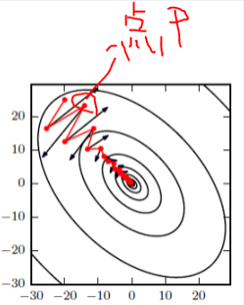如图所示，红色为SGD+Momentum。黑色为SGD。可以看到黑色为典型Hessian矩阵病态的情况，相当于大幅度的徘徊着向最低点前进。
而由于动量积攒了历史的梯度，如点P前一刻的梯度与当前的梯度方向几乎相反。因此原本在P点原本要大幅徘徊的梯度，主要受到前一时刻的影响，而导致在当前时刻的梯度幅度减小。
直观上讲就是，要是当前时刻的梯度与历史时刻梯度方向相似，这种趋势在当前时刻则会加强；要是不同，则当前时刻的梯度方向减弱。
2.算法实现
算法原理：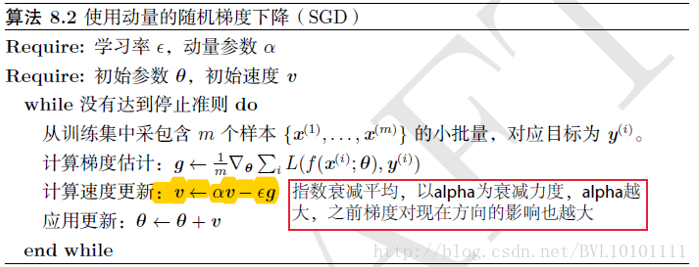pytorch-SGD+Momentum源码：
class SGD(Optimizer):
.... #函数注释

def __init__(self, params, lr=required, momentum=0, dampening=0,
weight_decay=0, nesterov=False):
... #初始化

def __setstate__(self, state):
super(SGD, self).__setstate__(state)
for group in self.param_groups:
group.setdefault('nesterov', False)

def step(self, closure=None):
"""Performs a single optimization step.

Arguments:
closure (callable, optional): A closure that reevaluates the model
and returns the loss.
"""
loss = None
if closure is not None:
loss = closure()

for group in self.param_groups:
weight_decay = group['weight_decay']
momentum = group['momentum'] 	#动量参数α
dampening = group['dampening']
nesterov = group['nesterov']

for p in group['params']:
continue
if weight_decay != 0:
d_p.add_(weight_decay, p.data)# d_p = d_p + (weight_decay * p)
if momentum != 0:
param_state = self.state[p]	#存放辅助性的参数
if 'momentum_buffer' not in param_state:
buf = param_state['momentum_buffer'] = torch.zeros_like(p.data)	#开辟内存空间，存放动量参数
buf.mul_(momentum).add_(d_p)# buf = buf * momentum + d_p
else:
buf = param_state['momentum_buffer']
buf.mul_(momentum).add_(1 - dampening, d_p)# buf = momentum * buf + (1 - dampening) * d_p
if nesterov:
d_p = d_p.add(momentum, buf)# d_p = d_p + momentum * buf
else:
d_p = buf

p.data.add_(-group['lr'], d_p)# p = p - lr * d_p

return loss
展开全文• 动量算法改进的BP算法matlab仿真实例，经验证。证 明一个3层的BP网络能够实现任意的连续映射,可以任意 精度逼近任何给定的连续函数 。
• Bkprop Köhnəproqramlarınyenihəyatı。 BkpropС＃-da。C#
• 动量法 使用梯度下降法，每次都会朝着目标函数下降最快的方向，这也称为最速下降法。这种更新方法看似非常快，实际上存在一些问题。 1. 梯度下降法的问题 考虑一个二维输入，[x1,x2][x_1, x_2][x1​,x2​]，输出的...
动量法
使用梯度下降法，每次都会朝着目标函数下降最快的方向，这也称为最速下降法。这种更新方法看似非常快，实际上存在一些问题。
1. 梯度下降法的问题
考虑一个二维输入，

[

x

1

,

x

2

]

[x_1, x_2]

，输出的损失函数

L

:

R

2

→

R

L: R^2 \rightarrow R

，下面是这个函数的等高线：可以想象成一个很扁的漏斗，这样在竖直方向上，梯度就非常大，在水平方向上，梯度就相对较小，所以我们在设置学习率的时候就不能设置太大，为了防止竖直方向上参数更新太过了，这样一个较小的学习率又导致了水平方向上参数在更新的时候太过于缓慢，所以就导致最终收敛起来非常慢。
2. 动量法
动量法的提出就是为了应对这个问题，我们梯度下降法做一个修改如下：

v

i

=

γ

v

i

−

1

+

η

∇

L

(

θ

)

v_i = \gamma v_{i-1} + \eta \nabla L(\theta)

θ

i

=

θ

i

−

1

−

v

i

\theta _i = \theta_{i-1} - v_i

其中

v

i

v_i

是当前速度，

γ

\gamma

是动量参数，是一个小于 1的正数，

η

\eta

是学习率
相当于每次在进行参数更新的时候，都会将之前的速度考虑进来，每个参数在各方向上的移动幅度不仅取决于当前的梯度，还取决于过去各个梯度在各个方向上是否一致，如果一个梯度一直沿着当前方向进行更新，那么每次更新的幅度就越来越大，如果一个梯度在一个方向上不断变化，那么其更新幅度就会被衰减，这样我们就可以使用一个较大的学习率，使得收敛更快，同时梯度比较大的方向就会因为动量的关系每次更新的幅度减少，如下图比如我们的梯度每次都等于 g，而且方向都相同，那么动量法在该方向上使参数加速移动，有下面的公式：

v

0

=

0

v_0 = 0

v

1

=

γ

v

0

+

η

g

=

η

g

v_1 = \gamma v_0 + \eta g = \eta g

v

2

=

γ

v

1

+

η

g

=

(

1

+

γ

)

η

g

v_2 = \gamma v_1 + \eta g = (1 + \gamma) \eta g

v

3

=

γ

v

2

+

η

g

=

(

1

+

γ

+

γ

2

)

η

g

v_3 = \gamma v_2 + \eta g = (1 + \gamma + \gamma^2) \eta g

⋯

\cdots

v

+

∞

=

(

1

+

γ

+

γ

2

+

γ

3

+

⋯

)

η

g

=

1

1

−

γ

η

g

v_{+ \infty} = (1 + \gamma + \gamma^2 + \gamma^3 + \cdots) \eta g = \frac{1}{1 - \gamma} \eta g

如果我们把

γ

\gamma

定为 0.9，那么更新幅度的峰值就是原本梯度乘学习率的 10 倍。
本质上说，动量法就仿佛我们从高坡上推一个球，小球在向下滚动的过程中积累了动量，在途中也会变得越来越快，最后会达到一个峰值，对应于我们的算法中就是，动量项会沿着梯度指向方向相同的方向不断增大，对于梯度方向改变的方向逐渐减小，得到了更快的收敛速度以及更小的震荡。
下面我们手动实现一个动量法，公式已经在上面了:
def sgd_momentum(parameters, vs, lr, gamma):
for param, v in zip(parameters, vs):
v[:] = gamma * v + lr * param.grad.data
param.data = param.data - v

import numpy as np
import torch
from torchvision.datasets import MNIST
import torch.nn as nn
import torch.nn.functional as F

import time
import matplotlib.pyplot as plt
%matplotlib inline

def data_tf(x):
x = np.array(x, dtype='float32') / 255
x = (x - 0.5) / 0.5 # 标准化，这个技巧之后会讲到
x = x.reshape((-1,)) # 拉平
x = torch.from_numpy(x)
return x

# 使用MNIST数据集，没有的话需要下载
train_set = MNIST('./data', train=True, transform=data_tf) # 载入数据集，申明定义的数据变换
test_set = MNIST('./data', train=False, transform=data_tf)

# 定义 loss 函数
criterion = nn.CrossEntropyLoss()

# DataLoader 对数据进行加载打包
# 使用 Sequential 定义 3 层神经网络
net = nn.Sequential(
nn.Linear(784, 200),
nn.ReLU(),
nn.Linear(200, 10),
)

# 将速度初始化为和参数形状相同的零张量
vs = []
for param in net.parameters():
vs.append(torch.zeros_like(param.data))

# 开始训练
losses = []

start = time.time() # 记时开始
for e in range(5):
train_loss = 0
for im, label in train_data:

# 前向传播
out = net(im)
loss = criterion(out, label)
# 反向传播
loss.backward()
sgd_momentum(net.parameters(), vs, 1e-2, 0.9) # 使用的动量参数为 0.9，学习率 0.01
# 记录误差
train_loss += loss.item()

losses.append(loss.item())
print('epoch: {}, Train Loss: {:.6f}'
.format(e, train_loss / len(train_data)))
end = time.time() # 计时结束
print('使用时间: {:.5f} s'.format(end - start))

epoch: 0, Train Loss: 0.363505
epoch: 1, Train Loss: 0.173747
epoch: 2, Train Loss: 0.124349
epoch: 3, Train Loss: 0.101248
epoch: 4, Train Loss: 0.084900
使用时间: 21.00233 s

可以看到，加完动量之后 loss 能下降非常快，但是一定要小心学习率和动量参数，这两个值会直接影响到参数每次更新的幅度，所以可以多试几个值
当然，pytorch 内置了动量法的实现，非常简单，直接在torch.optim.SGD(momentum=0.9) 即可，下面实现一下
train_data = DataLoader(train_set,batch_size = 64,shuffle=True)

# 使用nn.Module定义3层网络

class my_net(nn.Module):
def __init__(self):
super(my_net,self).__init__()
self.L1 = nn.Linear(784,200)
self.L2 = nn.Linear(200,10)

def forward(self,x):
x = F.relu(self.L1(x))
x = F.relu(self.L2(x))
return x

net=my_net()
print(net)

my_net(
(L1): Linear(in_features=784, out_features=200, bias=True)
(L2): Linear(in_features=200, out_features=10, bias=True)
)

import torch.optim as optim
optimizer=optim.SGD(net.parameters(),lr=1e-2,momentum=0.9)

criterion = nn.CrossEntropyLoss()

## 开始训练
losses = []
idx=0
start = time.time()# 计时开始
for e in range(5):
train_loss=0
for im,label in train_data:
# 前向传播
out = net(im)
loss = criterion(out,label)

# 反向传播
loss.backward()
optimizer.step()

# 记录误差
train_loss += loss.item()
if idx%30==0: # 30步记录一次
losses.append(loss.item())
idx += 1

print(f'epoch:{e},Train Loss:{train_loss/len(train_data):.6f}')
end = time.time()
print(f'使用时间：{end-start:.6f}s')

epoch:0,Train Loss:0.253888
epoch:1,Train Loss:0.236733
epoch:2,Train Loss:0.221444
epoch:3,Train Loss:0.206973
epoch:4,Train Loss:0.193939
使用时间：19.242222s

x_axis = np.linspace(0, 5, len(losses), endpoint=True)
plt.semilogy(x_axis, losses, label='momentum: 0.9')
plt.legend(loc='best')

<matplotlib.legend.Legend at 0x19ab8e64808>我们可以对比一下不加动量的随机梯度下降法
# 使用 Sequential 定义 3 层神经网络
net = nn.Sequential(
nn.Linear(784, 200),
nn.ReLU(),
nn.Linear(200, 10),
)

optimizer = torch.optim.SGD(net.parameters(), lr=1e-2) # 不加动量
# 开始训练
losses1 = []
idx = 0
start = time.time() # 记时开始
for e in range(5):
train_loss = 0
for im, label in train_data:

# 前向传播
out = net(im)
loss = criterion(out, label)
# 反向传播
loss.backward()
optimizer.step()
# 记录误差
train_loss += loss.item()
if idx % 30 == 0: # 30 步记录一次
losses1.append(loss.item())
idx += 1
print('epoch: {}, Train Loss: {:.6f}'
.format(e, train_loss / len(train_data)))
end = time.time() # 计时结束
print('使用时间: {:.5f} s'.format(end - start))

epoch: 0, Train Loss: 0.748911
epoch: 1, Train Loss: 0.366224
epoch: 2, Train Loss: 0.320932
epoch: 3, Train Loss: 0.294529
epoch: 4, Train Loss: 0.272778
使用时间: 18.94132 s

补充：格式化输出
a= 1.23333233313
print(‘a:{:.6f}’.format(a))print(f’a:{a:.6f}’) 两种输出形式是一样的
x_axis = np.linspace(0, 5, len(losses), endpoint=True)
plt.semilogy(x_axis, losses, label='momentum: 0.9')
plt.semilogy(x_axis, losses1, label='no momentum')
plt.legend(loc='best')

<matplotlib.legend.Legend at 0x19aba401048>可以看到加完动量之后的 loss 下降的程度更低了，可以将动量理解为一种惯性作用，所以每次更新的幅度都会比不加动量的情况更多。
参考：PyTorch中文手册
展开全文深度学习 神经网络
• 关于梯度下降的三个算法：梯度下降，随机梯度下降，以及小批量皮杜下降已经在该篇博文中介绍：https://blog.csdn.net/weixin_42109859/article/details/104822335 但对梯度下降使用同一固定不变的超参数会引发一些...
关于梯度下降的三个算法：梯度下降，随机梯度下降，以及小批量皮杜下降已经在该篇博文中介绍：https://blog.csdn.net/weixin_42109859/article/details/104822335 但对梯度下降使用同一固定不变的超参数会引发一些问题。 例如当我们的目标函数为

f

(

x

)

=

0.1

x

1

2

+

2

x

2

2

f(\boldsymbol{x})=0.1x_1^2+2x_2^2

，学习率采用0.4时，其损失的迭代轨迹如下：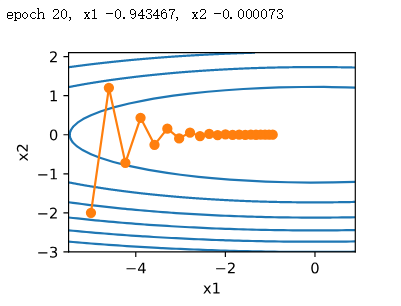可以看到，同一位置上，目标函数在竖直方向（

x

2

x_2

轴方向）比在水平方向（

x

1

x_1

轴方向）的斜率的绝对值更大。因此，给定学习率，梯度下降迭代自变量时会使自变量在竖直方向比在水平方向移动幅度更大（由于x2的系数为2造成竖直方向更新更快）。那么，我们需要一个较小的学习率从而避免自变量在竖直方向上越过目标函数最优解。然而，这会造成自变量在水平方向上朝最优解移动变慢。 若将学习率调的稍大，则此时自变量在竖直方向不断越过最优解并逐渐发散。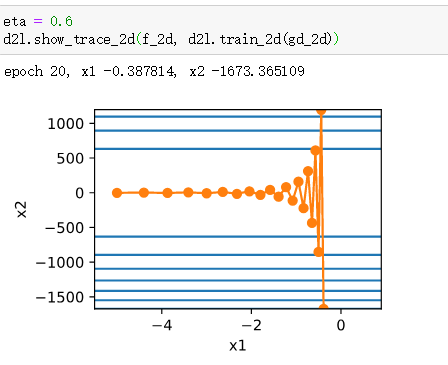动量法
基于指数加权移动平均的思想，提出了动量法予以解决上述问题。设时间步

t

t

的自变量为

x

t

\boldsymbol{x}_t

，学习率为

η

t

\eta_t

。 在时间步

0

0

，动量法创建速度变量

v

0

\boldsymbol{v}_0

，并将其元素初始化成0。在时间步

t

>

0

t>0

，动量法对每次迭代的步骤做如下修改：

v

t

←

γ

v

t

−

1

+

η

t

g

t

,

x

t

←

x

t

−

1

−

v

t

,

\begin{aligned} \boldsymbol{v}_t &\leftarrow \gamma \boldsymbol{v}_{t-1} + \eta_t \boldsymbol{g}_t, \\ \boldsymbol{x}_t &\leftarrow \boldsymbol{x}_{t-1} - \boldsymbol{v}_t, \end{aligned}

其中，动量超参数

γ

\gamma

满足

0

≤

γ

<

1

0 \leq \gamma < 1

。当

γ

=

0

\gamma=0

时，动量法等价于小批量随机梯度下降。 其效果如下：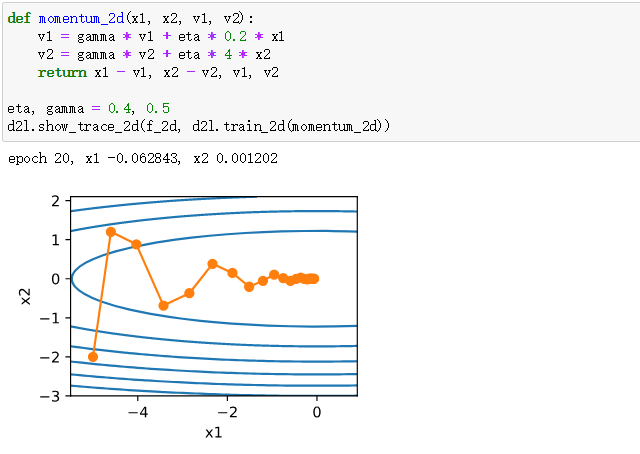可以观察出动量法使得相邻时间步的自变量更新在方向上更加一致。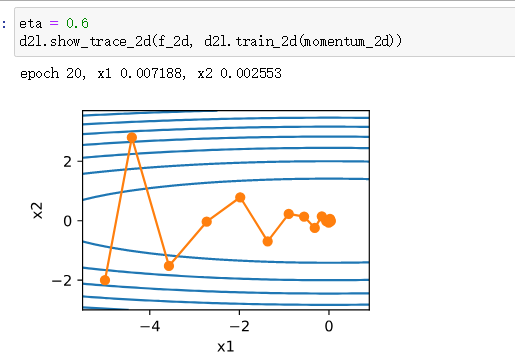当学习率较大时，其仍然收敛的较好且不发散。 我们先将动量超参数momentum设0.5，这时可以看成是特殊的小批量随机梯度下降：其小批量随机梯度为最近2个时间步的2倍小批量梯度的加权平均。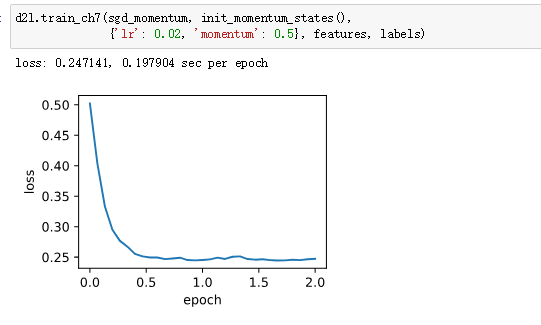将动量超参数momentum增大到0.9，这时依然可以看成是特殊的小批量随机梯度下降：其小批量随机梯度为最近10个时间步的10倍小批量梯度的加权平均。我们先保持学习率0.02不变。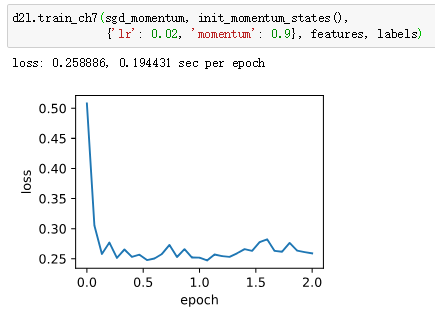总结： 动量法使用了指数加权移动平均的思想。它将过去时间步的梯度做了加权平均，且权重按时间步指数衰减。 动量法使得相邻时间步的自变量更新在方向上更加一致。
展开全文深度学习 人工智能 随机梯度下降
• Pytorch Note16 优化算法2 动量法(Momentum) 文章目录Pytorch Note16 优化算法2 动量法(Momentum)动量法(Momentum)梯度下降法的问题MomentumNesterov Accelerated Gradient代码从0实现pytorch 内置优化器对比 动量 +...深度学习 神经网络 人工智能 pytorch
• 周志华机器学习BP改进 ...- 启发式算法：如附加动量法，自适应算法 - 数值优化法：如共轭梯度法、牛顿迭代法、Levenberg-Marquardt算法 (1)附加动量项 这是一种广泛用于加速梯度下降法收敛...
• 来源：https://kexue.fm/archives/5655 目录 梯度下降 训练目标分析 GD与ODE 随机梯度下降 从GD到SGD ...动量加速  ...Nesterov动量 ...在本文中，我们来关心优化算法 SGD（stochastic gradient ...
• 动量梯度下降算法训练BP网络 使用的主要函数如下： NEWFF——生成一个新的前向神经网络 TRAIN——对BP神经网络进行训练 SIM——对BP神经网络进行仿真
• 我们表明，通过开发稀疏动量算法，我们可以使用稀疏随机权重初始化神经网络，并将其训练为密集的性能水平-所有这些都仅需执行一次训练即可。此外，如果我们使用优化的稀疏卷积算法，则可以将VGG的训练速度提高到3.5...
• 假如梯度一直都是g，那么会一直在-g上不停加速，直到达到最终速度，其中步长变为，如当a=0.9时对应最大速度十倍于梯度下降算法。实际中，一开始是一个较小的值。 同时，使用动量以后，会累积之前的梯度，假如梯度...
• ## 优化算法总结

千次阅读 2019-10-24 10:23:52
好久就想写一篇有关优化算法的文章，今天下定决心一定要把优化算法弄的明明白白。 一、梯度 想学习优化算法首先就要知道什么是梯度。 1、微积分中：梯度表示函数增长最快的方向。神经网络中：采用负梯度表示目标...神经网络 人工智能 机器学习
• 上篇文章介绍了指数加权平均，这篇文章介绍在此基础上介绍一下动量梯度下降算法。 所谓动量梯度下降算法，简言之就计算梯度的指数加权平均，然后使用这个梯度来更新权重，下面我们来详细解释这句话。 我们在使用...深度学习 机器学习 人工智能 神经网络
• 从形式上看，动量算法引入了变量v 充当速度角色——它代表参数在参数空间移动的方向和速率。速度被设为负梯度的指数衰减平均. 3. RMSprop RMSProp 算法缩放每个参数反比于其所有梯度历史平方值总和的平方根...优化 RMSprop adam momentum
• 此文章记录动量算法的原理，以及使用它的目的。 N.B. 此处的梯度下降算法是广义的，包括一般我们使用的是mini-batch SGD。 梯度下降：GD, SGD, mini-batch SGD 在理解动量之前，我们有必要先了解梯度下降算法随机...深度学习 机器学习
• clear all;  pause(1);  P=[0.1452 0.1466 0.1314 0.2243 0.3523 0.4642 0.5015 0.6981 0.7821 0.8345 0.9649 1.156 0.2415 0.3027 0;  0.1217 0.1581 0.1408 0.2304 0.3143 0.4312 0.5819 0.7125 0.8065 0....网络 图形 测试
• 该算法通过在粒子群算法中引入一种新的二进制交叉策略来加快粒子群算法的收敛速度, 通过设置新的惯性权重来改善新算法的全局搜索和局部搜索能力, 并且在粒子搜索路径中引入变系数低通滤波器构成的动量算法来平滑粒子...
• 该算法通过在粒子群算法中引入一种新的二进制交叉策略来加快粒子群算法的收敛速度, 通过设置新的惯性权重来改善新算法的全局搜索和局部搜索能力, 并且在粒子搜索路径中引入变系数低通滤波器构成的动量算法来平滑粒子...
• ## 深度学习中的动量

千次阅读 2018-06-08 16:35:23
动量算法积累了之前梯度指数级衰减的移动平均，并且继续沿该方向移动。 动量的效果如下图所示。 动量的主要目的是解决两个问题： Hessian 矩阵的病态条件和随机梯度的方差。我们通过此图说明动量如何克服这两...
• ## 随机梯度下降与动量详解

千次阅读 多人点赞 2018-05-23 15:18:33
1. SGD公式理解 注：这一部分引用自知乎用户Qi Qi，原回答链接 随机梯度下降主要用来求解类似于如下求和形式的优化问题： ...普通梯度下降算法： wt+1=wt−ηt+1∇f(wt)=wt−ηt+1∑i=1n∇fi(wt,xi...
• 从形式上看， 动量算法引入了变量 z充当速度角色——它代表参数在参数空间移动的方向和速率。速度被设为负梯度的指数衰减平均。名称 动量（momentum），相当于引入动量前的梯度概念，指示着loss在参数空间下一步要...
• 理解梯度下降法是理解动量梯度下降法的前提，除此之外要搞懂动量梯度下降法需要知道原始方法在实际应用中的不足之处，动量梯度下降法怎样改善了原来方法的不足以及其具体的实现算法。依次从以下几个方面进行说明： ...随机梯度下降法...